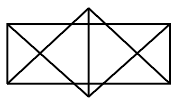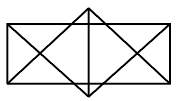Question 11

# How many triangles are there in the following figure?Solution

Given thatafter counting triangle then

total triangle = 24 Ans